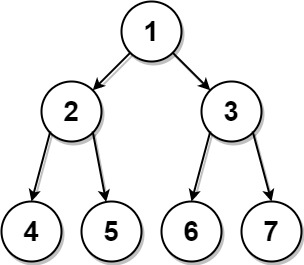0889. 根据前序和后序遍历构造二叉树

# 0889. 根据前序和后序遍历构造二叉树#

• 标签：树、数组、哈希表、分治、二叉树
• 难度：中等

## 题目大意 #

• $1 \le preorder.length \le 30$。
• $1 \le preorder[i] \le preorder.length$。
• preorder 中所有值都不同。
• postorder.length == preorder.length
• $1 \le postorder[i] \le postorder.length$。
• postorder 中所有值都不同。
• 保证 preorderpostorder 是同一棵二叉树的前序遍历和后序遍历。

• 示例 1：1 2  输入：preorder = [1,2,4,5,3,6,7], postorder = [4,5,2,6,7,3,1] 输出：[1,2,3,4,5,6,7] 
• 示例 2：
 1 2  输入: preorder = , postorder =  输出:  

## 解题思路 #

### 思路 1：递归 #

1. 从前序遍历序列中可知当前根节点的位置在 preorder

2. 前序遍历序列的第 2 个值为左子树的根节点，即 preorder。通过在后序遍历中查找上一步根节点对应的位置 postorder[k]（该节点右侧为右子树序列），从而将二叉树的左右子树分隔开，并得到左右子树节点的个数。

3. 从上一步得到的左右子树个数将后序遍历结果中的左右子树分开。

4. 构建当前节点，并递归建立左右子树，在左右子树对应位置继续递归遍历并执行上述三步，直到节点为空。

### 思路 1：代码 #

  1 2 3 4 5 6 7 8 9 10 11 12 13 14 15  class Solution: def constructFromPrePost(self, preorder: List[int], postorder: List[int]) -> TreeNode: def createTree(preorder, postorder, n): if n == 0: return None node = TreeNode(preorder) if n == 1: return node k = 0 while postorder[k] != preorder: k += 1 node.left = createTree(preorder[1: k + 2], postorder[: k + 1], k + 1) node.right = createTree(preorder[k + 2: ], postorder[k + 1: -1], n - k - 2) return node return createTree(preorder, postorder, len(preorder)) 

### 思路 1：复杂度分析 #

• 时间复杂度：$O(n^2)$。其中 $n$ 是二叉树的节点数目。
• 空间复杂度：$O(n^2)$。